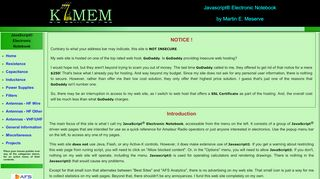### Single-Layer Air-CoreHits## Single-Layer Air-Core

A diagram of the coil and the basic equation used for the calculations are shown in the diagramCalculate Radio Frequency Power Density
This is a main beam power density estimation program intended for use as part of a routine evaluation of RFCalculate the frequency/length of antennas, KB4SAI
Frequency in Mhz, half Wavelength in Feet, half Wavelength in Inches, quarter Wavelength Feet, quarter Wavelength in InchesCalculate your dipole - 3 element yagi, W4HN
Frequency in MHz, 1/4 wave , MHz, 1/2 wave, 7/8 wave , full waveCalculation dipole inverterv
Formula, 468 / f MHz to calculate dipole lengthsCalculation program 10GHz waveguide slot antenna, DK3BA,DH6SBN